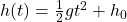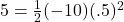## An observer standing near a window 5 m high observe that an object falling downwards is passing across the window in 0.5 s. Find at what hei

Question

An observer standing near a window 5 m high observe that an object falling downwards is passing across the window in 0.5 s. Find at what height above the window the object was dropped. (g = 10 m/s2).​

in progress 0
2 months 2021-07-23T06:11:42+00:00 1 Answers 67 views 0

Explanation:

An object in free fall, NOT experiencing parabolic motion, has an equation ofwhich says:

The height of an object with respect to time in seconds is equal to the pull of gravity times time-squared plus the height from which it was dropped. Normally we use -9.8 for gravity but you said to use 10, so be it.

For us, h(t) is 5 because we are looking for the height of the window when the object is 5 m off the ground at .5 seconds;

g = 10 m/s/s, and

t = .5sec+h and

5 = -5(.5)² + h and

5 = -5(.25) + h and

5 = -1.25 + h so

h = 6.25

That’s how high the window is above the ground.